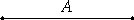# Proposition 33

If a number has its half odd, then it is even-times-odd only.

Let the number A have its half odd.

I say that A is even-times-odd only.VII.Def.9

Now that it is even-times-odd is clear, for the half of it, being odd, measures it an even number of times.

I say next that it is also even-times-odd only.

VII.Def.8

If A is even-times even also, then it is measured by an even number according to an even number, so that the half of it is also measured by an even number though it is odd, which is absurd.

Therefore A is even-times-odd only.

Therefore, if a number has its half odd, then it is even-times-odd only.

Q.E.D.

## Guide

To say that a number is even-times-odd only means that it is even-times-odd, but it is not even-times-even. As this proposition states, such numbers are the numbers which are twice odd numbers.